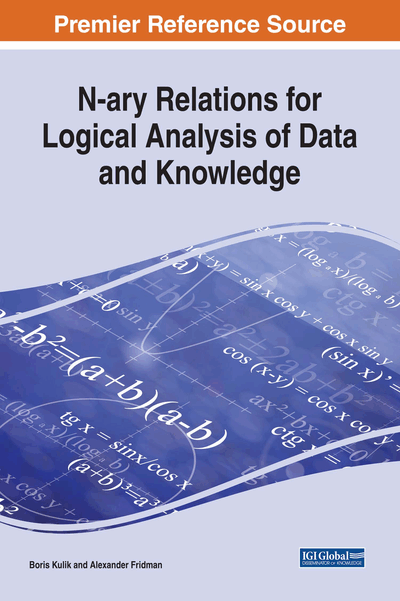# Deductive Analysis in NTA

Copyright: © 2018 |Pages: 23
DOI: 10.4018/978-1-5225-2782-4.ch003
OnDemand PDF Download:
Available
\$37.50
No Current Special Offers

## Abstract

This chapter mostly describes implementation of logical inference by means of NTA. Besides the known logical calculus methods, NTA logical inference procedures can include new algebraic methods for checking correctness of a consequence or for finding corollaries to a given axiom system. Above feasibility of certain substitutions, these inference methods consider inner structure of knowledge to be processed thus providing faster solving of standard logical analysis tasks.
Chapter Preview

You can really admire an original idea only if you know deduction, since it is the difference that counts. – Anonymous Logician

Top

## Nta As An Interpretation Of Logical Calculi

Consider a system which will help us to understand that NTA can serve an algebraic interpretation of some well-known logical calculi.

Assume a system M is given with the set of possible states Y = {t1, t2, ..., tr}. M includes a plurality X of nodes or subsystems X = {X1, X2, ..., Xn}. In turn, each node Xi can have a set of states {,, ...,} where ki is the number of such states.

The exact model of M is given by a correspondence between all possible sets of states for all nodes and the system states. A set of elementary tuples from the Cartesian product D = X1 × X2 × ... × Xn corresponds to any system state tjY. The mathematical scheme of this correspondence is:

M: DY. (1)

At first sight, the model (1) loses its practical significance with growing number of nodes, as well as with increasing number of states for nodes and the system itself, since even in relatively small systems, the number of sets of elementary states may exceed capabilities of modern computers. However, calculi usually exploit models in which, firstly, the number of system states is relatively small (for classical logic, they are only 2, namely true and false) and, secondly, every system state comprises such sets of options for nodes states in which only some subsets of nodes are used. In this case, the states of other nodes do not affect the state of the system. For instance, the state t2 of a system may correspond to a relation defined in the space X2 × X4 × X5, and other nodes (subsystems) of the universe D have no effect on the mentioned state. This can significantly reduce the amount of source data for such models.

To clarify the relationships between NTA and different variants of calculi, we introduce several limitations, which can be considered in the subject area for its representation by the model (1).

## Complete Chapter List

Search this Book:
Reset